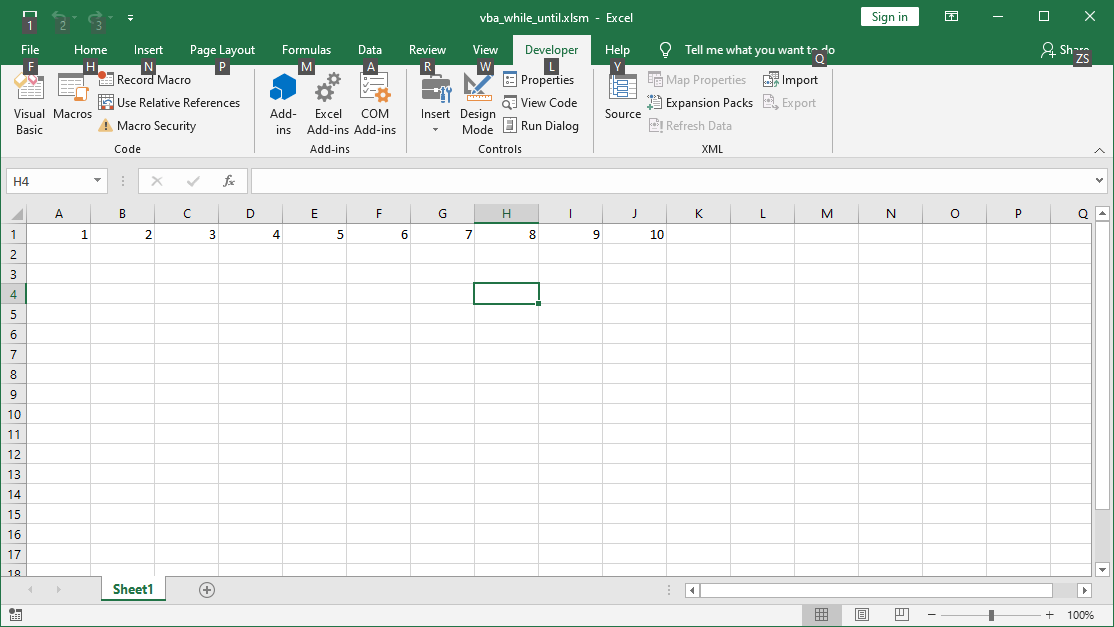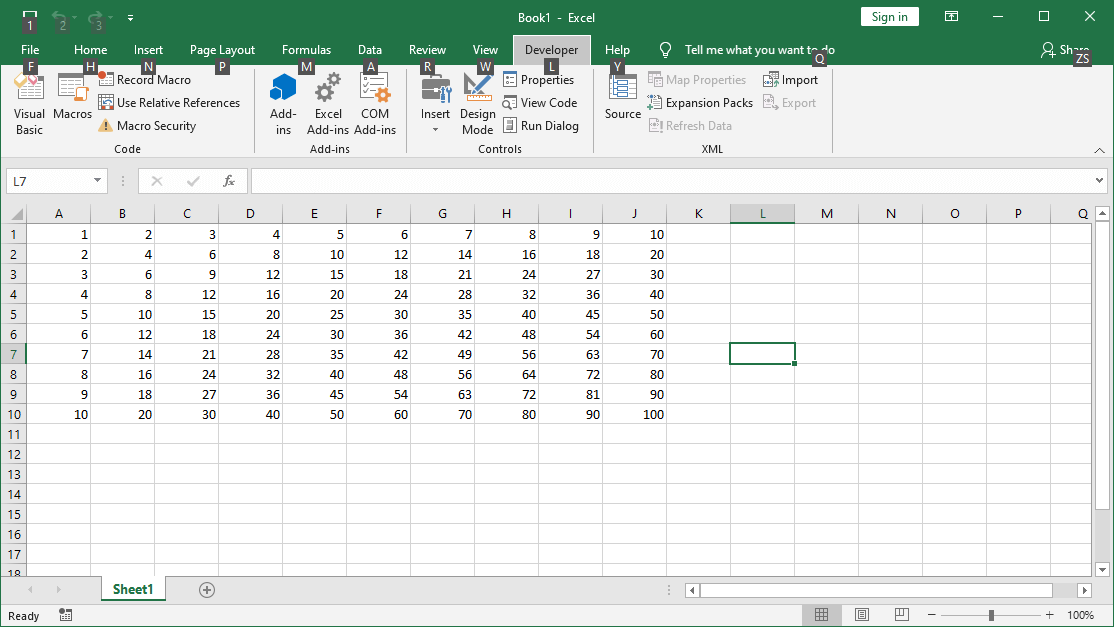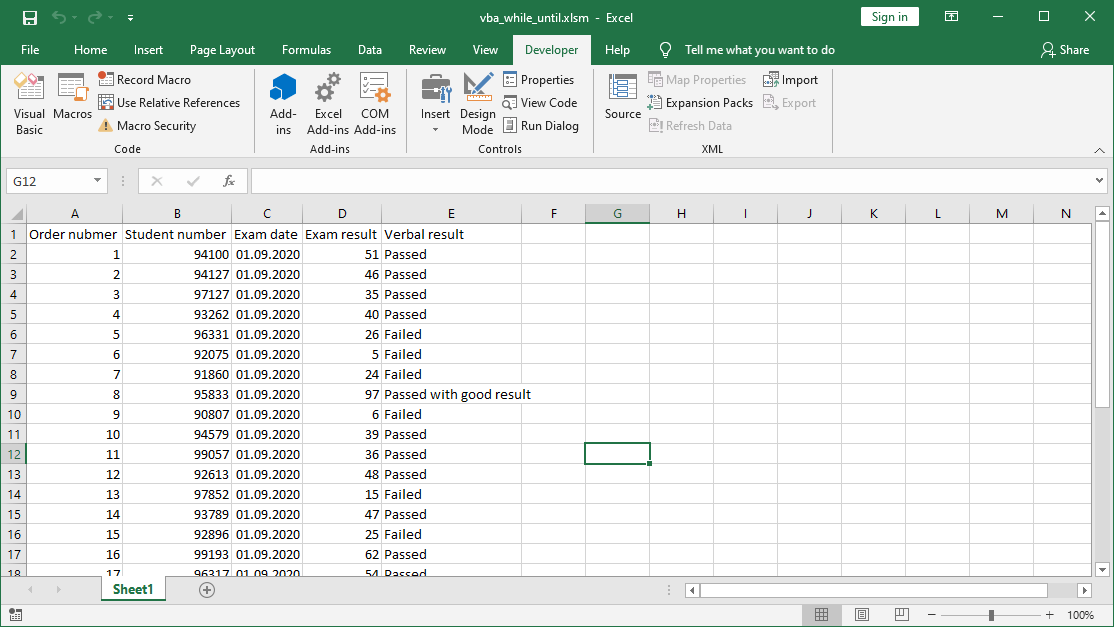# VBA Do While Loop and Do Until Loop

## The diagram of Do While Loop

The While…Wend loop, which you learned in the previous chapter is the simplest loop in the Excel VBA. The loop that gives you more options is Do Loop. It gives you more possibilities. You can, for example, finish the instructions earlier, by entering the exit condition. Your control over the execution of instructions is much greater. Below is the basic scheme of Do…Loop statement:

```Do [Condition type] [Conditions]
[Code to execute]
Loop```

## VBA Do Loop types – Do While and Do Until

Do Loop can be group in 5 categories. Description of them you can see in this table:

You can terminate loop before the condition is True. The Exit Do statement is used for this. You use it, for example, if you want to put an additional condition to finish the loop. A condition that you didn’t write after While or Until.

## Do While Loop and Do Until Loop Examples

Let’s try to create a simple table with VBA code. We will use the Do While LoopDo Until Loop and Do loop Until loops we’ve learned for before. First, let’s create a simple loop that fills 10 columns of the first row in our sheet using Do While Loop:

```Sub LoopExample1()
Dim intRow As Integer
Dim intCol As Integer
intRow = 1
intCol = 1
Do While intCol <= 10
Cells(intRow, intCol) = intCol * intRow
intCol = intCol + 1
Loop
End Sub```

You can do the same operation using Do Until Loop:

```Sub LoopExample2()

Dim intRow As Integer
Dim intCol As Integer
intRow = 1
intCol = 1

Do
Cells(intRow, intCol) = intCol * intRow
intCol = intCol + 1
Loop Until intCol = 10

End Sub```Do While Loop example and Do Until Loop example

## VBA – An example of a loop in another loop

In the examples, we intentionally introduced both variables for columns and rows. We will use them fully ending our example. Once we have the macro filling the first row, we loop it, increasing the counter for the rows by 1 each time. In this way, a multiplication table up to 100 will be created in your sheet.

```Sub LoopExample3()

Dim intRow As Integer
Dim intCol As Integer
intRow = 1

Do Until intRow > 10
intCol = 1

Do
Cells(intRow, intCol) = intCol * intRow
intCol = intCol + 1
Loop Until intCol > 10

intRow = intRow + 1
Loop

End Sub```VBA Do While multiplication table up to 100

## How to create simple table with data using Do While Loop or Do Until Loop?

In the example, you will create a simple table of people data. After creating the table, we will deal with the data analysis using the previously known functions and capabilities of VBA. For starters, let’s create a simple table, in which columns will be:

• Order number,
• student number,
• exam date,
• exam result,
• verbal result.

Additionally, the sequence number column (order number) should be filled in with numbers from 1 to 30.

```Sub LoopExample()

Dim intCounter As Integer
Dim intColumn As Integer
Dim intRow As Integer

'colmn names
Cells(1, 1) = "Order nubmer"
Cells(1, 2) = "Student number"
Cells(1, 3) = "Exam date"
Cells(1, 4) = "Exam result"
Cells(1, 5) = "Verbal result"

'order numbers from 1 to 30
intCounter = 1
Do While intCounter <= 30
Cells(intRow + 2, 1) = intCounter
intCounter = intCounter + 1
intRow = intRow + 1
Loop

End Sub```How to create simple table with data using Do While Loop or Do Until Loop?

In this way we have obtained a simple table to which we can enter data. Next, using the function of generating random numbers, modify our script so that:

• The Index number column fill with random numbers between 90000 and 100000
• The date of the exam column fill with the date of today – 10 days
• The result of the exam contained random numbers from 1 to 100
• The data contained in the Verbal result column should refer to the results as follows: results from 0 to 33 are given the “Failed” value. Values ​​from 34 to 67 receive the value “Pass.” Values ​​greater than 67 receive the value “Pass with good result”.

Random numbers are created using the VBA RND function . We can generate random numbers from 0 to 1 in this way. The number can be converted into integers and round using the VBA INT function . These functions are described in more detail in the article on the VBA mathematical functions.

```Sub LoopExample5()
Dim intCounter As Integer
Dim intColumn As Integer
Dim intRow As Integer

'column names
Cells(1, 1) = "Order nubmer"
Cells(1, 2) = "Student number"
Cells(1, 3) = "Exam date"
Cells(1, 4) = "Exam result"
Cells(1, 5) = "Verbal result"

'order numbers from 1 to 30
intCounter = 1
Do While intCounter <= 30
Cells(intRow + 2, 1) = intCounter
intCounter = intCounter + 1
intRow = intRow + 1
Loop

'student number using RND function
intCounter = 1 'set counter 1
intRow = 0 'set row counter 0
Do While intCounter <= 30
Cells(intRow + 2, 2) = Int(90000 + Rnd() * 10000) 'random value from 90k to 100k
intCounter = intCounter + 1
intRow = intRow + 1
Loop

'exam date column
intCounter = 1 'set counter 1
intRow = 0 'set row counter 0
Do While intCounter <= 30
Cells(intRow + 2, 3) = Date - 10 ' dates in 3rd column
intCounter = intCounter + 1
intRow = intRow + 1
Loop

'exam result column
intCounter = 1 'set counter 1
intRow = 0 'set row counter 0
Do While intCounter <= 30
Cells(intRow + 2, 4) = Int(Rnd() * 100) 'multiplication random value by 100 and round
intCounter = intCounter + 1
intRow = intRow + 1
Loop

'verbal result - result in words
Dim StrResult As String 'var for result in words
intCounter = 1 'set counter 1
intRow = 0 'set row counter 0
Do While intCounter <= 30

'Verbal result
If Cells(intRow + 2, 4) <= 33 Then
StrResult = "Failed"
ElseIf Cells(intRow + 2, 4) > 33 And Cells(intRow + 2, 4) <= 67 Then
StrResult = "Passed"
ElseIf Cells(intRow + 2, 4) > 67 Then
StrResult = "Passed with good result"
End If

Cells(intRow + 2, 5) = StrResult

intCounter = intCounter + 1
intRow = intRow + 1
Loop

End Sub```How to create simple table with data using Do While Loop or Do Until Loop?

The file with code:

## Tasks (You can enter the solution in a comment)

1. Create a multiplication table using the Do While Loop.
2. Using a loop, list all even numbers from 0 to 100 in the sheet.
3. Use the loops to list all numbers between 0 and 100, divisible by 4.
4. Modify the example with the people database by adding a color in column 5. Depending on the result of the exam, the result of the pass corresponds to yellow, the result corresponds to failed will be red. For the result he passed good result assign green color.